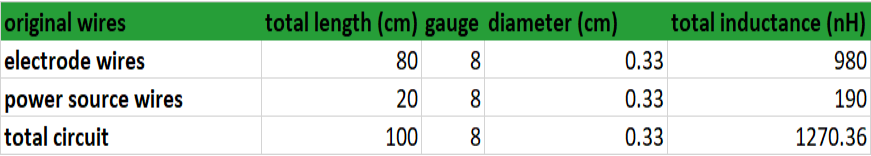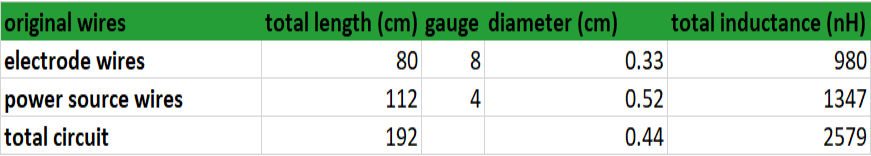inductance, Q&A for kWeld, wire gauge, wire gauge to diameter, wire length -

Can I change the wire gauge or length on my kWeld spot welder?

Calculating the inductance of the kWeld circuit

Your kWeld spot welder came with a set of stock wires which many are tempted to change. We encourage the DIY mentality and love the fact that you rewire masters share your mods and experiences with the community on kWeld World.

Firstly, please understand that too much inductance can damage the kWeld unit. Increasing the wire length and related inductance also reduces the maximum current your kWeld can handle safely. Hence, in the setup, the wire length is entered in order for the software to limit the maximum current and protect the kWeld spot welder.

In the manuals, Frank at keenlab discusses the inductance calculations at length. Here, we’ll try to summarize the impact of changing wires with one example. We will also link the related charts and an on-line inductance calculator so that you can explore the impact of your desired changes.

In this example, the kWeld is powered by a 12V deep cycle lead acid battery. It is capable of 1000CCA. The rewire master in this example wanted to lengthen the power cables using 4 AWG cables measuring 56cm each (22”).In this chart, you will notice that the total circuit inductance in not equal to the addition of the two smaller circuits. This complicates the calculation for a change of only the power supply cables since the new cables are a different gauge. The method used in this following chart was to average the wire diameter out over the entire circuit to find a value for comparison.

Each wire is added in this manner: (8AWG) 40cm + 40cm = 80cm, (4AWG) 56cm + 56cm = 112cm, Total wire length is 192cm. The 8AWG wire has a diameter of 0.33cm, the 4AWG wire has a diameter of 0.52cm. The average diameter over the full length of wire is 0.44cm.You can see that this change more than doubles the total inductance in the kWeld circuit.

In order for you to calculate inductance for your desired change, we include here and on our resource page, some links to the resources we used for these calculations.

Inductance calculator

Submit your question so that we can build a useful Q&A

info@gridrewired.com

Español